Students can Download Tamil Nadu 12th Physics Model Question Paper 5 English Medium Pdf, Tamil Nadu 12th Physics Model Question Papers helps you to revise the complete Tamilnadu State Board New Syllabus and score more marks in your examinations.

## TN State Board 12th Physics Model Question Paper 5 English Medium

Instructions:

1. The question paper comprises of four parts
2. You are to attempt all the parts. An internal choice of questions is provided wherever: applicable
3. All questions of Part I, II, III and IV are to be attempted separately
4. Question numbers 1 to 15 in Part I are Multiple choice Questions of one mark each.  These are to be answered by choosing the most suitable answer from the given four alternatives and writing the option code and the corresponding answer
5. Question numbers 16 to 24 in Part II are two-mark questions. These are lo be answered in about one or two sentences.
6. Question numbers 25 to 33 in Part III are three-mark questions. These are lo be answered in about three to five short sentences.
7. Question numbers 34 to 38 in Part IV are five-mark questions. These are lo be answered in detail. Draw diagrams wherever necessary.

Time: 3 Hours
Max Marks: 70

PART – 1

Answer all the questions. Choose the correct answer. [15 × 1 = 15]

Question 1.
When the current changes from +2A to -2A in 0.05 s, an emf of 8 V is induced in a coil. The co-efficient of self-induction of the coil is
(a) 0.2 H
(b) 0.4 H
(c) 0.8 H
(d) 0.1 H
(d) 0.1 HQuestion 2.
If λv , λx, λm, represent the wavelength of visible light, X-rays and microwaves, respectively, then
(a) λm > λv > λx
(b) λm > λx > λv
(c) λv > λm > λx
(d) λv > λx > λm
(a) λm > λv > λx

Question 3.
The materials used in Robotics are:
(a) Aluminium and silver
(b) silver and gold
(c) Copper and gold
(d) Steel and aluminium
(d) Steel and aluminium

Question 4.
Two wires of A and B with circular cross-section are made up of the same material with equal lengths. If RA = 3RB, then what is the ratio of radius of wire A to that of B?
(a) 3
(b) √3
(c) $$\frac{1}{\sqrt{3}}$$
(d) $$\frac{1}{3}$$
(c) $$\frac{1}{\sqrt{3}}$$

Question 5.
The frequency range of 3 MHz to 30 MHz is used for
(a) Ground wave propagation
(b) Space wave propagation
(c) Sky wave propagation
(d) Satellite communication
(c) Sky wave propagation

Question 6.
A ray of light strikes a glass plate at an angle 60°. If the reflected and refracted rays are perpendicular to each other, the refractive index of the glass is,
(a) √3
(b) $$\frac{3}{2}$$
(c) $$\sqrt{\frac{3}{2}}$$
(d) 2
Hint. Angle of refraction r = 60° ; Angle of incident i = 30°
sin i = n × sin r
n = $$\frac{\sin 30^{\circ}}{\sin 60^{\circ}}=\sqrt{3}$$
(a) √3

Question 7.
If voltage applied on a capacitor is increased from V to 2V choose the correct conclusion
(a) Q remains the same, C is doubled
(b) Q is doubled, C doubled
(c) C remains same, Q doubled
(d) Both Q and C remain same
(c) C remains same, Q doubled

Question 8.
The nucleus is approximately spherical in shape. Then the surface area of the nucleus having mass number A varies as
(a) A2/3
(b) A4/3
(c) A1/3
(d) A5/3
Hint. Volume of nucleus is proportional to mass number 4
$$\frac { 4 }{ 3 }$$ π R3 ∝ A : R = R0 A1/3
So, 4 π R2 = R0 A2/3 ⇒ 4 π R2 ∝ A2/3
Surface area is proportional to (mass number)23
(a) A2/3Question 9.
The given electrical network is equivalent to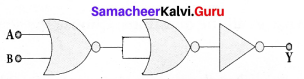(a) AND gate
(b) OR gate
(c) NOR gate
(d) NOT gate
(c) NOR gate

Question 10.
A wire of length / carries a current I along the Y direction and magnetic field is given by $$\vec{B}$$ = $$\frac{\beta}{\sqrt{3}}$$(i +j +k)T- The magnitude of Lorentz force acting on the wire is
(a) $$\sqrt{\frac{2}{\sqrt{3}}} \beta I l$$
(b) $$\sqrt{\frac{1}{\sqrt{3}}} \beta \mathrm{Il}$$
(c) $$\sqrt{2} \beta \mathrm{Il}$$
(d) $$\sqrt{\frac{1}{2}} \beta \mathrm{Il}$$
(a) $$\sqrt{\frac{2}{\sqrt{3}}} \beta I l$$

Question 11.
When a point charge of 6 pC is moved between two points in an electric field, the work done is 1.8 × 10-5 J. This potential difference between the two points is :
(a) 1.08 V
(b) 1.08 μV
(c) 3 V
(d) 30 V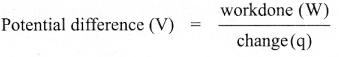$$=\frac{1.8 \times 10^{-5}}{6 \times 10^{-6}}=\frac{18}{6}$$
V = 3V
(c) 3 V

Question 12.
The wavelength λe of an electron and λp of a photon of same energy E are related by
(a) λp ∝ λe
(b) λp ∝ $$\sqrt{\lambda_{e}}$$
(c) λp ∝ $$\frac{1}{\sqrt{\lambda_{e}}}$$
(d) λp ∝ λe2
Hint:
de-Breglie wavelength of proton λe = $$\frac{h}{\sqrt{2 m \mathrm{E}}}$$
∴ ie λe ∝ $$\frac{1}{\sqrt{\mathrm{E}}}$$ ⇒ λe2 ∝ $$\frac{1}{E}$$ …….. (1)
de-Breglie wave length of proton
λp = $$\frac{h c}{E}$$
λp ∝ $$\frac{1}{E}$$ …….. (2)
From (1) and (2)
λe2 ∝ λp i.e., λp ∝ λe2
(d) λp ∝ λe2

Question 13.
For a myopic eye, the defect is cured by using a:
(a) convex lens
(b) concave lens
(c) cylindrical lens
(d) plane glass
(b) concave lensQuestion 14.
In a tangent galvanometer experiment, for two different values of current if the deflection are 45° and 30° respectively, then the ratio of the current is:
(a) 2 : 3
(b) 3 : 2
(c) √3 : 1
(d) 1 : √3
Hint: I1 = tan θ1
I2 = tan θ2
$$\frac{I_{1}}{I_{2}}=\frac{\tan 45^{\circ}}{\tan 30^{\circ}}=\frac{1}{\left(\frac{1}{\sqrt{3}}\right)}=\frac{\sqrt{3}}{1}$$
(c) √3 : 1

Question 15.
If the current gain a of a transistor is 0.98, what is the value of p of the transistor?
(a) 0.49
(b) 49
(c) 4.9
(d) 5
Hint: $$\beta=\frac{\alpha}{1-\alpha}=\frac{0.98}{1-0.98}=49$$
(b) 49

PART – II

Answer any six questions in which Q. No 24 is compulsory. [6 × 2 = 12]

Question 16.
What is meant by Fraunhofer lines?
When the spectrum obtained from the Sun is examined, it consists of large number of dark lines (line absorption spectrum). These dark lines in the solar spectrum are known as Fraunhofer lines.

Question 17.
Why steel is preferred in making Robots?
Steel is several time stronger. In any case, because of the inherent strength of metal, robot bodies are made using sheet, bar, rod, channel, and other shapes.

Question 18.
State Lenz’s law.
Lenz’s law states that the direction of the induced current is such that it always opposes the cause responsible for its production.

Question 19.
Why do clouds appear white?
Clouds have large particles like dust and water droplets which scatter light of all colours almost equally. Hence clouds generally appear white.Question 20.
Calculate the radius of 19779Au nucleus.
According to the equation R = R0A1/3
R = 1.2 × 10-15 × (197)1/3 = 6.97 × 10-15 m (or) R = 6.97 F

Question 21.
What is the need for a feedback circuit in a transistor oscillator?
The circuit used to feedback a portion of the output to the input is called the feedback network. If the portion of the output fed to the input is in phase with the input, then the magnitude of the input signal increases. It is necessary for sustained oscillations.

Question 22.
Show graphically the variation of electric held £ (y-axis) due to a charged infinite plane sheet with distance r (x-axis) from the plate.
It independent of the distance. It straight line parallel to x-axis.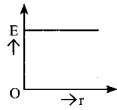Question 23.
Give any two applications of internet.
(i) Search engine: The search engine is basically a web-based service tool used to search for information on World Wide Web.

(ii) Communication: It helps millions of people to connect with the use of social networking: emails, instant messaging services and social networking tools.

(iii) E-Commerce: Buying and selling of goods and services, transfer of funds are done over an electronic network.

Question 24.
Calculate the magnetic field inside a solenoid when the number of turns is halved and the length of the solenoid and the area remain the same.
Magnetic field inside a solenoid is B = $$\frac{\mu_{0} \mathrm{NI}}{\mathrm{L}}$$
If the number of turns is havled B’ = $$\mathrm{B}^{\prime}=\frac{\mu_{0}\left(\frac{\mathrm{N}}{2}\right) \mathrm{I}}{\mathrm{L}} \Rightarrow \mathrm{B}^{\prime}=\frac{1}{2}\left(\frac{\mu_{0} \mathrm{NI}}{\mathrm{L}}\right)$$PART – III

Answer any six questions in which Q.No. 33 is compulsory. [6 × 3 = 18]

Question 25.
Two cells each of 5V are connected in series across a 8 12 resistor and three parallel resistors of 4 12, 6 12 and 12 12. Draw a circuit diagram for the above arrangement calculate
(i) the current drawn from the cell
(ii) current through each resistor.
V1 = 5 V; V2 = 5 v
R1 = 8 Ω; R2 = 4Ω; R3 = 6Ω; R4 = 12Ω
Three resistors R2, R3 and R4 are connected parallel combination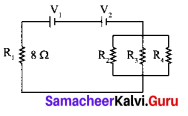$$\frac{1}{R_{p}}=\frac{1}{R_{2}}+\frac{1}{R_{3}}+\frac{1}{R_{4}}$$
$$=\frac{1}{4}+\frac{1}{6}+\frac{1}{12}=\frac{3}{12}+\frac{2}{12}+\frac{1}{12}=\frac{6}{12}$$
Rp = 2Ω
Resistors R1 and Rp are connected in series combination
Rs = R1 + Rp = 8 + 2 = 10
R2 = 10Ω
Total voltage connected series to the circuit
V = V1 + V2
= 5 + 5 =10
V = 10V

(i) Current through the circuit, I = $$\frac{V}{R_{s}}=\frac{10}{10}$$
I = IA
Potential drop across the parallel combination,
V’ = I Rp = 1 × 2 .
V’ = 2 V

(ii) Current in 4Ω resistor, I = $$\frac{V^{\prime}}{R_{2}}=\frac{2}{4}$$ = 0.5 A
Current in 6Ω resistor, I = $$\frac{V^{\prime}}{R_{3}}=\frac{2}{6}$$ = 0.33 A.
Current in 12Ω resistor, I = $$\frac{V^{\prime}}{R_{4}}=\frac{2}{12}$$ = 0.17 A

Question 26.
Explain the various energy losses in a transformer.
Energy losses in a’ transformer: Transformers do not have any moving parts so that its efficiency is much higher than that of rotating machines like generators and motors. But there are many factors which lead to energy loss in a transformer.

1. Core loss or Iron loss: This loss takes place in transformer core. Hysteresis loss and eddy current loss are known as core loss or Iron loss. When transformer core is magnetized and demagnetized repeatedly by the alternating voltage applied across primary coil, hysteresis takes place due to which some energy is lost in the form of heat. Hysteresis loss is minimized by using steel of high silicon content in making transformer core. Alternating magnetic flux in the core induces eddy currents in it. Therefore there is energy loss due to the flow of eddy current, called eddy current loss which is minimized by using very thin laminations of transformer core.

2. Copper loss: Transformer windings have electrical resistance. When an electric current flows through them, some amount of energy is dissipated due to Joule heating. This energy loss is called copper loss which is minimized by using wires of larger diameter.

3. Flux leakage: Flux leakage happens when the magnetic lines of primary coil are not completely linked with secondary coil. Energy loss due to this flux leakage is minimized by winding coils one over the other.Question 27.
Discuss the alpha – decay process with example.
When unstable nuclei decay by emitting an α-particle (42He nucleus), it loses two protons and two neutrons. As a result, its atomic number Z decreases by 2, the mass number decreases by 4. We write the alpha decay process symbolically in the following way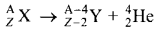Here X is called the parent nucleus and Y is called the daughter nucleus.
Example: Decay of Uranium 23892U to thorium 23490Th with the emission of 42He nucleus (α-particle)
23892U → 23490Th + 42He
As already mentioned, the total mass of the daughter nucleus and 2 He nucleus is always less than that of the parent nucleus. The difference in mass ( Δm = mx – my mα) is released as energy called disintegration energy Q and is given by
Q = (mx – my – mα) c2
Note that for spontaneous decay (natural radioactivity) Q > 0. In alpha decay process, the disintegration energy is certainly positive (Q > 0). In fact, the disintegration energy Q is also the net kinetic energy gained in the decay process or if the parent nucleus is at rest, Q is the total kinetic energy of daughter nucleus and the 42He nucleus. Suppose Q < 0, then the decay process cannot occur spontaneously and energy must be supplied to induce the decay.

Question 28.
Obtain the expression for energy stored in the parallel plate capacitor.
Energy stored in the capacitor: Capacitor not only stores the charge but also it stores energy. When a battery is connected to the capacitor, electrons of total charge -Q are transferred from one plate to the other plate. To transfer the charge, work is done by the battery. This work done is stored as electrostatic potential energy in the capacitor.
To transfer an infinitesimal charge dQ for a potential difference V, the work done is given by
dW = VdQ ….(1)
Where V = $$\frac{Q}{C}$$
The total work done to charge a capacitor is
W = $$\int_{0}^{0} \frac{\mathrm{Q}}{\mathrm{C}} d \mathrm{Q}=\frac{\mathrm{Q}^{2}}{2 \mathrm{C}}$$ ….. (2)
This work done is stored as electrostatic potential energy (UE) in the capacitor.
UE = $$\frac{\mathrm{Q}^{2}}{2 \mathrm{C}}=\frac{1}{2} \mathrm{CV}^{2}$$ (∴Q = CV) …… (3)
where Q = CV is used. This stored energy is thus directly proportional to the capacitance of the capacitor and the square of the voltage between the plates of the capacitor. But where is this energy stored in the capacitor? To understand this question, the equation (3) is rewritten as follows using the results
C = $$\frac{\varepsilon_{0} A}{d}$$ and V = Ed
UE = $$\frac{1}{2}\left(\frac{\varepsilon_{0} \mathrm{A}}{d}\right)(\mathrm{E} d)^{2}=\frac{1}{2} \varepsilon_{0}(\mathrm{A} d) \mathrm{E}^{2}$$ …… (4)
where Ad = volume of the space between the capacitor plates. The energy stored per unit Volume of space is defined as energy density uE = $$\frac{\mathrm{U}}{\text { Volume }}$$ From equation (4) we get
UE = $$\frac{1}{2} \varepsilon_{0} \mathrm{E}^{2}$$ ……. (5)
From equation (5), we infer that the energy is stored in the electric field existing between the plates of the capacitor. Once the capacitor is allowed to discharge, the energy is retrieved.

Question 29.
Explain any three recent advancements in medical technology.
1. Virtual reality:
Medical virtual reality is effectively used to stop the brain from processing pain and cure soreness in the hospitalized patients. Virtual reality has enhanced surgeries by the use of 3D models by surgeons to plan operations. It helps in the treatment of Autism, Memory loss, and Mental illness.

2. Precision medicine:
Precision medicine is an emerging approach for disease treatment and prevention that takes into account individual variability in genes, environment, and lifestyle for each person. In this medical model it is possible to customise healthcare, with medical decisions, treatments, practices, or products which are tailored to the individual patient.

3. Health wearables:
A health wearable is a device used for tracking a wearer’s vital signs or health and fitness related data, location, etc. Medical wearables with artificial intelligence and big data provide an added value to healthcare with a focus on diagnosis, treatment, patient monitoring and prevention.
Note: Big Data: Extremely large data sets that may be analysed computationally to reveal patterns, trends, and associations, especially relating to human behaviour and interactions.Question 30.
Two light sources with amplitudes 5 units and 3 units respectively interfere with each other. Calculate the ratio of maximum and minimum intensities.
Amplitudes, a1 = 5, a2 = 3
Resultant amplitude, A = $$\sqrt{a_{1}^{2}+a_{2}^{2}+2 a_{1} a_{2} \cos \phi}$$
Resultant amplitude is maximum when,
Φ = 0, cos 0 = 1, Amax = $$\sqrt{a_{1}^{2}+a_{2}^{2}+2 a_{1} a_{2}}$$
Amax = $$\sqrt{\left(a_{1}+a_{2}\right)^{2}}=\sqrt{(5+3)^{2}}=\sqrt{(8)^{2}}$$ = 8 units
Resultant amplitude is minimum when, Φ = π, cos π = -1, Amax = $$\sqrt{a_{1}^{2}+a_{2}^{2}-2 a_{1} a_{2}}$$
Amin = $$\sqrt{\left(a_{1}-a_{2}\right)^{2}}=\sqrt{(5-3)^{2}}=\sqrt{(2)^{2}}$$ = 2 units
I ∝ A2
$$\frac{I_{\max }}{I_{\min }}=\frac{\left(A_{\max }\right)^{2}}{\left(A_{\min }\right)^{2}}$$
Substituting, $$\frac{I_{\max }}{I_{\min }}=\frac{(8)^{2}}{(2)^{2}}=\frac{64}{4}=16$$ (or) Imax : Imin = 16 : 1

Question 31.
An electron moves in a circular orbit with a uniform speed v it produces a magnetic field B at the centre of the circle. Prove that the radius of the circle is proportional to $$\sqrt{\frac{v}{\mathbf{B}}}$$
The magnetic field produce by moving electron in circular path.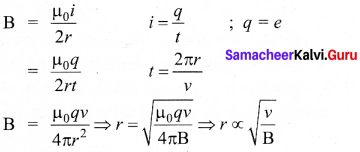Question 32.
Give the construction and working of photo emissive cell.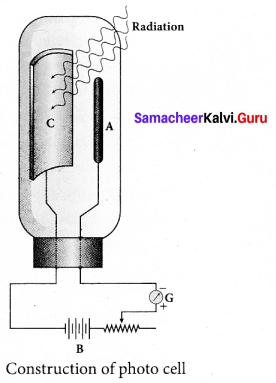Photo emissive cell:
Its working depends on the electron emission from a metal cathode due to irradiation of light or other radiations.
Construction:

• It consists of an evacuated glass or quartz bulb in which two metallic electrodes – that is, a cathode and an anode are fixed.
• The cathode C is semi-cylindrical in shape and is coated with a photo sensitive material. The anode A is a thin rod or wire kept along the axis of the semi-cylindrical cathode.
• A potential difference is applied between the anode and the cathode through a galvanometer G.

Working:

• When cathode is illuminated, electrons are emitted from it. These electrons are attracted by anode and hence a current is produced which is measured by the galvanometer.
• For a given cathode, the magnitude of the current depends on
• the intensity to incident radiation and
• the potential difference between anode and cathode.Question 33.
In the circuit shown in the figure the input voltage V1 = +5 V, VBE = + 0.8 V and VCE = + 0.12 VCE. Find the values of IB, IC and β.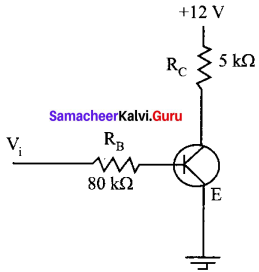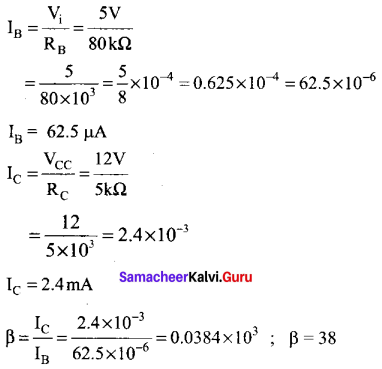PART – IV

Answer all the questions. [5 × 5 = 25]

Question 34.
(a) Obtain the expression for electric field due to an uniformly charged spherical shell at a distance its centre.
Electric field due to a uniformly charged spherical shell: Consider a uniformly charged spherical shell of radius R and total charge Q. The electric field at points outside and inside the sphere is found using Gauss law.

Case (a) At a point outside the shell (r > R): Let us choose a point P outside the shell at a distance r from the center as shown in figure (a). The charge is uniformly distributed on the surface of the sphere (spherical symmetry). Hence the electric field must point radially outward if Q > 0 and point radially inward if Q < 0. So we choose a spherical Gaussian surface of radius r and the total charge enclosed by this Gaussian surface is Q. Applying Gauss law,
$$\oint \overrightarrow{\mathrm{E}} \cdot d \overrightarrow{\mathrm{A}}=\frac{\mathrm{Q}}{\varepsilon_{0}}$$ …(1)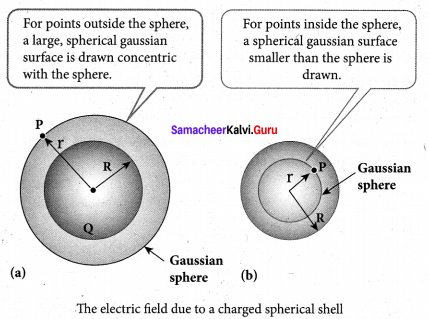The electric field $$\vec{E}$$ and $$d \vec{A}$$ point in the same direction (outward normal) at all the points on the Gaussian surface. The magnitude of $$\vec{E}$$ is also the same at all points due to the spherical symmetry of the charge distribution.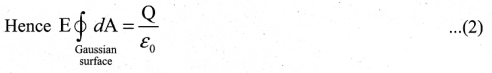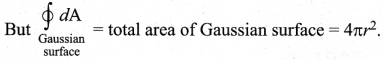Substituting this value in equation (2).
E.4πr2 = $$\frac{Q}{\varepsilon_{0}}$$
E.4πr2 = $$\frac{Q}{\varepsilon_{0}}$$ (or) E = $$\frac{1}{4 \pi \varepsilon_{0}} \frac{Q}{r^{2}}$$
In vector form $$\overrightarrow{\mathrm{E}}=\frac{1}{4 \pi \varepsilon_{0}} \frac{\mathrm{Q}}{r^{2}} \hat{r}$$

The electric field is radially outward if Q > 0 and radially inward if Q < 0. From equation (3), we infer that the electric field at a point outside the shell will be same as if the entire charge Q is concentrated at the center of the spherical shell. (A similar result is observed in gravitation, for gravitational force due to a spherical shell with mass M)

Case (b): At a point on the surface of the spherical shell (r = R): The electrical field at points on the spherical shell (r = R) is given by
$$\overrightarrow{\mathrm{E}}=\frac{Q}{4 \pi \varepsilon_{0} R^{2}} \hat{r}$$ …… (4)

Case (c) At a point inside the spherical shell (r < R): Consider a point P inside the shell at a distance r from the center. A Gaussian sphere of radius r is constructed as shown in the figure (b). Applying Gauss law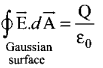E.4πr2 = $$\frac{Q}{\varepsilon_{0}}$$ ….. (5)
Since Gaussian surface encloses no charge, So Q = 0. The equation (5) becomes
E = 0 (r < R) …….. (6)
The electric field due to the uniformly charged spherical shell is zero at all points inside the shell.[OR]

Question 34.
(b) Write any five properties of electromagnetic waves.
Properties of electromagnetic waves:

1. Electromagnetic waves are produced by any accelerated charge.
2. Electromagnetic waves do not require any medium for propagation. So electromagnetic wave is a non-mechanical wave.
3. Electromagnetic waves are transverse in nature. This means that the oscillating electric field vector, oscillating magnetic field vector and propagation vector (gives direction of propagation) are mutually perpendicular to each other.
4. Electromagnetic waves travel with speed which is equal to the speed of light in vacuum, or freer space, c = $$\frac{1}{\sqrt{\varepsilon_{0} \mu_{0}}}$$ = 3 × 108 ms-1
5. The speed of electromagnetic wave is less than speed in free space or vacuum, that is, v < c. In a medium of refractive index,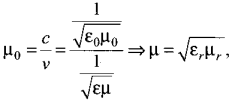Question 35.
(a) What is modulation? Explain the types of modulation with necessary diagrams.
Modulation: For long distance transmission, the low frequency baseband signal (input signal) is superimposed onto a high frequency radio signal by a process called modulation.
There are 3 types of modulation based on which parameter is modified. They are
(i) Amplitude modulation,
(ii) Frequency modulation, and
(iii) Phase modulation.

(i) Amplitude Modulation (AM):
If the amplitude of the carrier signal is modified according to the instantaneous amplitude of the baseband signal, then it is called amplitude modulation. Here the frequency and the phase of the carrier signal remain constant. Amplitude modulation is used in radio and TV broadcasting.

The signal shown in figure (a) is the message signal or baseband signal that carries information, figure (b) shows the high frequency carrier signal and figure (c) gives the amplitude modulated signal. We can see clearly that the carrier wave is modified in proportion to the amplitude of the baseband signal.
Amplitude Modulation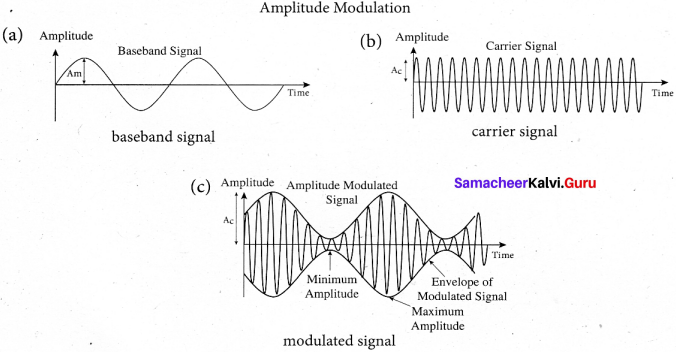(ii) Frequency Modulation (FM):
The frequency of the camer signal is modified according to the instantaneous amplitude of the baseband signal in frequency modulation. Here the amplitude and the phase of the carrier signal remain constant. Increase in the amplitude of the baseband signal increases the frequency of the carrier signal and vice versa. This leads to compressions and rarefactions in the frequency spectrum of the modulated wave. Louder signal leads to compressions and relatively weaker signals to rarefactions. When the amplitude of the baseband signal is zero in Figure (a), the frequency of the modulated signal is the same as the carrier signal. The frequency of the modulated wave increases when the amplitude of the baseband signal increases in the positive direction
(A, C). The increase in amplitude in the negative half cycle (B,D) reduces the frequency of the modulated wave (figure (c)).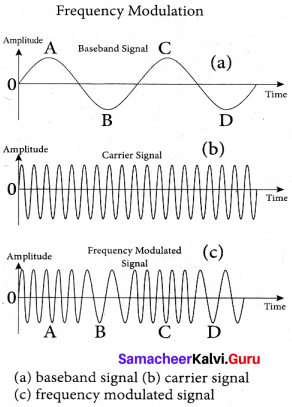(iii) Phase Modulation (PM):
The instantaneous amplitude of the baseband signal modifies the phase of the camer signal keeping the amplitude and frequency constant is called phase modulation. This modulation is used to generate frequency modulated signals. It is similar to frequency modulation except that the phase of the carrier is varied instead of varying frequency. The carrier phase changes according to increase or decrease in the amplitude of the baseband signal. When the modulating signal goes positive, the amount of phase
lead increases with the amplitude of the modulating signal. Due to this, the carrier signal is compressed or its frequency is increased.

On the other hand, the negative half cycle of the baseband signal produces a phase lag in the carrier signal. This appears to have stretched the frequency of the carrier wave. Hence similar to frequency modulated wave, phase modulated wave also comprises of
compressions and rarefactions. When the signal voltage is zero (A, C and E) the carrier frequency is unchanged.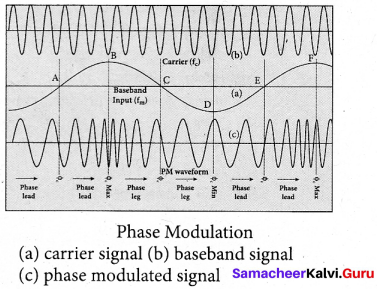[OR]Question 35.
(b) Find the expression for the mutual inductance between a pair of coils and show that (M12 = M21).
Mutual induction: When an electric current passing through a coil changes with time, an emf is induced in the neighbouring coil. This phenomenon is known as mutual induction and the emf is called mutually induced emf.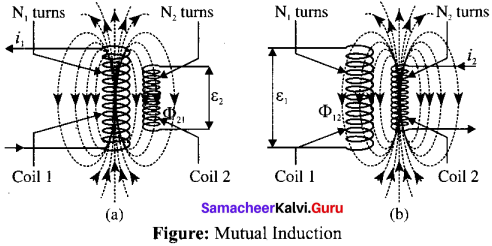Consider two coils which are placed close to each other. If an electric current q is sent through coil i1 the magnetic field produced by it is also linked with coil 2.
Let Φ21 be the magnetic flux linked with each turn of the coil 2 of N2 turns due to coil 1, then the total flux linked with coil 2 (N2Φ21) is proportional to the current i1 in the coil 1.
N2Φ21 ∝ i1
N2Φ21 = M21i1 or M21 = $$\frac{N_{2} \Phi_{21}}{i_{1}}$$
The constant of proportionality M21 is the mutual inductance of the coil 2 with respect to coil 1. It is also called as coefficient of mutual induction. If i1 = 1 A, then M21 = N2Φ21.
Therefore, the mutual inductance M21 is defined as the flux linkage of the coil 2 when 1A current flows through coil 1.
When the current i1 changes with time, an emf ξ2 is induced in coil 2. From Faraday’s law of electromagnetic induction, this mutually induced emf ξ21 is given by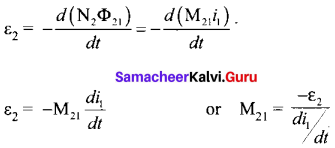The negative sign in the above equation shows that the mutually induced emf always opposes the change in current with respect to time. If $$\frac{d i_{1}}{d t}$$ = 1 As-1, then M21 = -ξ2.
Mutual inductance M21 is also defined as the opposing emf induced in the coil 2 when the rate of change of current through the coil I is l As-1.
Similarly, if an electric current i2 through coil 2 changes with time, then emf ξ1 is induced in coil 1. Therefore, N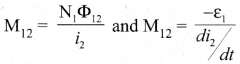where M12 is the mutual inductance of the coil I with respect to coil 2. It can be shown that for a given pair of coils, the mutual inductance is same. i.e., M21 = M12 = M.
In general, the mutual induction between two coils depends on size, shape, the number of turns of the coils, their relative orientation and permeability of the medium.Question 36.
(a) Derive the expression for the radius of the orbit of the electron and its velocity using Bohr atom model.
Radius of the orbit of the electron and velocity of the electron:
Consider an atom which contains the nucleus at rest and an electron revolving around the nucleus in a circular orbit of radius rn as shown in figure. Nucleus is made up of protons and neutrons. Since proton is positively charged and neutron is electrically neutral, the charge of a nucleus is purely the total charge of protons.

Let Z be the atomic number of the atom, then +Ze is the charge of the nucleus. Let -e be the charge of the electron. From Coulomb’s law, the force of attraction between the nucleus and the electron is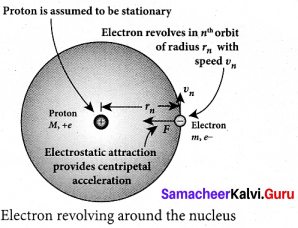$$\vec{F}$$coloumb = $$\frac{1}{4 \pi \varepsilon_{0}} \frac{(+\mathrm{Ze})(\mathrm{e})}{\mathrm{r}_{\mathrm{n}}^{2}} \hat{\mathrm{r}}$$
$$=-\frac{1}{4 \pi \varepsilon_{0}} \frac{Z e^{2}}{r_{n}^{2}} \hat{r}$$
This force provides necessary centripetal force
$$\vec{F}$$centripetal = $$\frac{m v_{n}^{2}}{r_{n}} \hat{r}$$
where m be the mass of the electron that moves with a velocity DW in a circular orbit. Therefore,
|$$\vec{F}$$coloumb| = |$$\vec{F}$$centripetal|
$$\frac{1}{4 \pi \varepsilon_{0}} \frac{Z e^{2}}{r_{n}^{2}}=\frac{m v_{n}^{2}}{r_{n}}$$
rn = $$\frac{4 \pi \varepsilon_{0}\left(m \mathrm{v}_{n} r_{n}\right) 2}{Z m e^{2}}$$ ……. (1)
From Bohr’s assumption, the angular momentum quantization condition mυnrn = ln = nn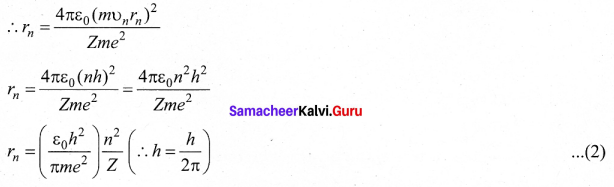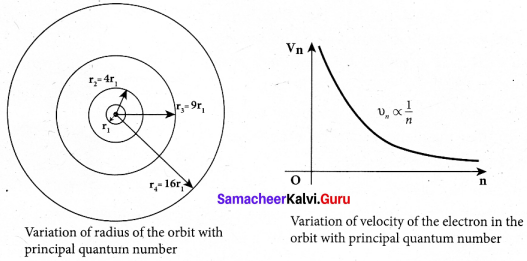where n ∈ N . Since, ε0, h, e and n are constants. Therefore, the radius of the orbit becomes
rn = a0$$\frac{n^{2}}{Z}$$
Where a0 = $$\frac{\varepsilon_{0} h^{2}}{\pi m e^{2}}$$ = 0.529Å. This is known as Bohr radius which is the smallest radius of the orbit in an atom. Bohr radius is also used as unit of length called Bohr. 1 Bohr = 0.53 Å. For hydrogen atom (Z = 1), the radius of nth orbit is
rn = a0n2
For the first orbit (ground state),
r1 = a0 = 0.529 Å
For the second orbit (first excited state),
r2 = 4a0 = 2.116 Å
For the third orbit (second excited state),
r3 = 9a0 = 4.761 Å and so on.
Thus the radius of the orbit from centre increases with n, that is, rn ∝ c n2 as shown in figure. Further, Bohr’s angular momentum quantization condition leads to
nrn = mυna0n2 = n$$\frac{h}{2 \pi}$$
υn = $$\frac{h}{2 \pi m a_{0}} \frac{Z}{n}$$
υn ∝ $$\frac{1}{n}$$
Note that the velocity of electron decreases as the principal quantum number increases as shown in figure. This curve is the rectangular hyperbola. This implies that the velocity of electron in ground state is maximum when compared to excited states.

[OR]Question 36.
(b) Discuss the working and theory of cyclotron in detail.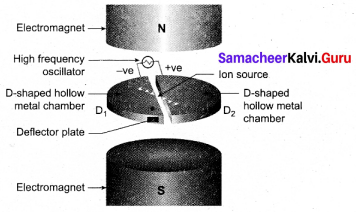Cyclotron: Cyclotron is a device used to accelerate the charged particles to gain large kinetic energy. It is also called as high energy accelerator. It was invented by Lawrence and Livingston in 1934.

Principle: When a charged particle moves normal to the magnetic field, it experiences magnetic Lorentz force.

Construction:The particles are allowed to move in between two semicircular metal containers called Dees (hollow D – shaped objects). Dees are enclosed in an evacuated chamber and it is kept in a region with uniform magnetic field controlled by an electromagnet. The direction of magnetic field is normal to the plane of the Dees. The two Dees are kept separated with a gap and the source S (which ejects the particle to be accelerated) is placed at the center in the gap between the Dees. Dees are connected to high frequency alternating potential difference.

Working: Let us assume that the ion ejected from source S is positively charged. As soon as ion is ejected, it is accelerated towards a Dee (say, Dee – 1) which has negative potential at that time. Since the magnetic field is normal to the plane of the Dees, the ion undergoes circular path. After one semi-circular path in Dee-1, the ion reaches the gap between Dees. At this time, the polarities of the Dees are reversed so that the ion is now accelerated towards Dee-2 with a greater velocity. For this circular motion, the centripetal force of the charged particle q is provided by Lorentz force.
$$\frac{m v^{2}}{r}$$ = qv B ⇒ r = $$\frac{m}{q \mathrm{B}} v$$ ⇒ r ∝ v
From the equation, the increase in velocity increases the radius of circular path. This process continues and hence the particle undergoes spiral path of increasing radius. Once it reaches near the edge, it is taken out with the help of deflector plate and allowed to hit the target T. Very important condition in cyclotron operation is the resonance condition. It happens when the frequency f at which the positive ion circulates in the magnetic field must be equal to the constant frequency of the electrical oscillator fosc From equation
fosc = $$\frac{q \mathbf{B}}{2 \pi m} \Rightarrow \mathrm{T}=\frac{1}{f_{\mathrm{osc}}}$$
The time period of oscillation is T = $$\frac{2 \pi m}{q B}$$
The kinetic energy of the charged particle is K E = $$\frac{1}{2} m v^{2}=\frac{q^{2} \mathbf{B}^{2} r^{2}}{2 m}$$
Limitations of cyclotron
(a) the speed of the ion is limited
(b) electron cannot be accelerated
(c) uncharged particles cannot be acceleratedQuestion 37.
(a) Obtain lens maker’s formula and mention its significance.
Lens maker’s formula and lens equation: Let us consider a thin lens made up of a medium of refractive index n2 is placed in a medium of refractive index n1 . Let R1 and R2 be the radii of curvature of two spherical surfaces (1) and (2) respectively and P be the pole. Consider a point object O on the principal axis. The ray which falls very close to P, after refraction at the surface (1) forms image at I’. Before it does so, it is again refracted by the surface (2). Therefore the final image is formed at I. The general equation for the refraction at a spherical surface is given by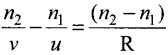For the refracting surface (1), the light goes from to n1 to n2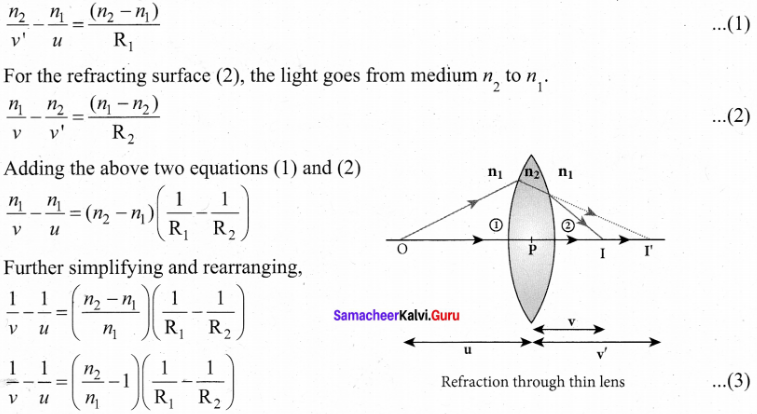If the object is at infinity, the image is formed at the focus of the lens. Thus, for u = oo, v =/ Then the equation becomes.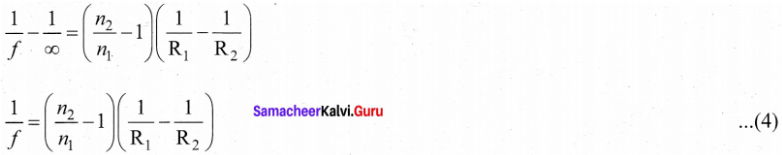If the refractive index of the lens is n2 and it is placed in air, then n2 = n and n1 = 1. So the equation (4) becomes,
$$\frac{1}{f}=(n-1)\left(\frac{1}{\mathrm{R}_{1}}-\frac{1}{\mathrm{R}_{2}}\right)$$

The above equation is called the lens maker’s formula, because it tells the lens manufactures what curvature is needed to make a lens of desired focal length with a material of particular refractive index. This formula holds good also for a concave lens. By comparing the equations (3) and (4) we can write,
$$\frac{1}{v}-\frac{1}{u}=\frac{1}{f}$$ ……… (6)
This equation is known as lens equation which relates the object distance it and image distance v with the focal length / of the lens. This formula holds good for a any type of lens.Question 37.
(b) Explain the construction and working of a full wave rectifier.
Full wave rectifier:
The positive and negative half cycles of the AC input signal pass through the full wave rectifier circuit and hence it is called the full wave rectifier. It consists of two p-n junction diodes, a center tapped transfonner, and a load resistor (RL). The centre is usually taken as the ground or zero voltage reference point. Due to the centre tap transformer, the output voltage rectified by each diode is only one half of the total secondary voltage.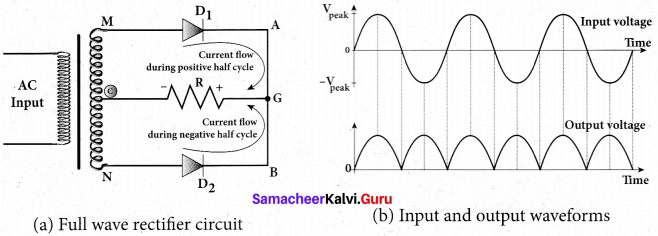During positive half cycle:
When the positive half cycle of the ac input signal passes through the circuit, terminal M is positive, G’ is at zero potential and N is at negative potential. This forward biases diode D1 and reverse biases diode D2 Hence, being forward biased, diode D1 conducts and current flows along the path MD1 AGC . As a result, positive half cycle of the voltage appears across RL in the direction G to C.

During negative half cycle:
When the negative half cycle of the ac input signal passes through the circuit, terminal N is positive, G is at zero potential and M is at negative potential. This forward biases diode D2 and reverse biases diode D1. Hence, being forward biased, diode D2 conducts and current flows along the path ND2 BGC . As a result, negative half cycle of the voltage appears across RL in the same direction from G to C

Hence in a full wave rectifier both positive and negative half cycles of the input signal pass through the circuit in the same direction as shown in figure (b). Though both positive and negative half cycles of ac input are rectified, the output is still pulsating in nature.

The efficiency ( η) of full wave rectifier is twice that of a half wave rectifier and is found to be 81.2 %. It is because both the positive and negative half cycles of the ac input source are rectified.Question 38.
(a) (i) Derive the expression for the de Broglie wavelength of an electron.
An electron of mass m is accelerated through a potential difference of V volt. The kinetic energy acquired by the electron is given by
$$\frac{1}{2} m v^{2}$$ = eV
Therefore, the speed v of the electron is v = $$\sqrt{\frac{2 e \mathrm{V}}{m}}$$
Hence, the de Broglie wavelength of the electron is λ = $$\frac{h}{m v}=\frac{h}{\sqrt{2 e m \mathrm{V}}}$$
Substituting the known values in the above equation, we get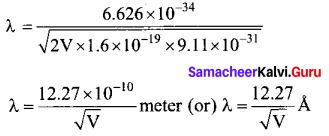For example, if an electron is accelerated through a potential difference of 100V, then its de Broglie wavelength is 1.227 Å . Since the kinetic energy of the electron, K = eV, then the de Broglie wavelength associated with electron can be also written as
λ = $$\frac{h}{\sqrt{2 m \mathrm{K}}}$$

(ii) An electron is accelerated through a potential difference of 81V. What is the de Broglie wavelength associated with it? To which part of the electromagnetic spectrum does this wavelength correspond?
de-Broglie wavelength of an electron beam accelerated through a potential difference of V volts is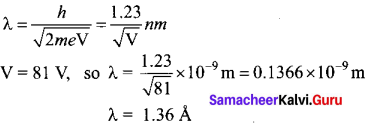X-ray is the part of electromagnetic spectrum does this wavelength corresponds. X-ray has the wavelengths ranging from about 10-8 to 10-12 m.[OR]

Question 38.
(b) (i) How will you measure the internal resistance of a cell by potentiometer?
Measurement of internal resistance of a cell by potentiometer:
To measure the internal resistance of a cell, the circuit connections are made as shown in figure. The end C of the potentiometer wire is connected to the positive terminal of the battery Bt and the negative terminal of the battery is connected to the end D through a key K1 This forms the primary circuit.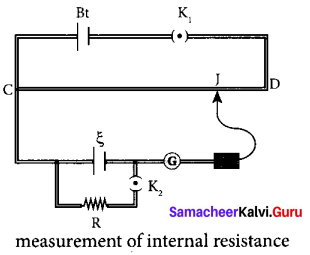The positive terminal of the cell ξ whose internal resistance is to be determined is also connected to the end C of the wire. The negative terminal of the cell ξ is connected to a jockey through a galvanometer and a high resistance. A resistance box R and key K2 are connected across the cell ξ. With K2 open, the balancing point J is obtained and the balancing length CJ = l1 is measured. Since the cell is in open circuit, its emf is
ξ ∝ l1 ….. (1)
A suitable resistance (say, 10 H) is included in the resistance box and key K2 is closed.
Let r be the internal resistance of the cell. The current passing through the cell and the resistance R is given by
I = $$\frac{\xi}{R+r}$$
The potential difference across R is
V = $$\frac{\xi R}{R+r}$$
When this potential difference is balanced on the potentiometer wire, let /2 be the balancing length.
Then $$\frac{\xi R}{R+r} \propto l_{2}$$ ….. (2)
From equations (1) and (2)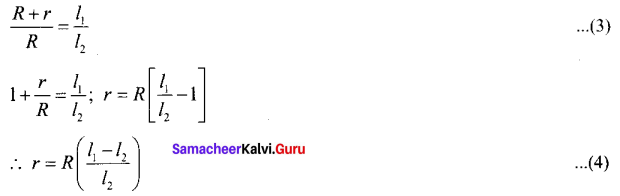Substituting the values of the R, l1 and l2, the internal resistance of the cell is determined. The experiment can be repeated for different values of R. It is found that the internal resistance of the cell is not constant but increases with increase of external resistance connected across its terminals.(ii) A cell supplies a current of 0.9 A through a 1Ω resistor and a current of 0.3 A through a 2 Ω resistor. Calculate the internal resistance of the cell.
Current from the cell 1,I1, = 0.9 A and Resistor, R1 = 1Ω
Current from the cell 2,I2 = 0.3 A and Resistor, R2 = 2Ω
Current in the circuit, I1 = $$\frac{\xi}{r+R_{1}}$$
ξ = I1r + I1R1 ….. (1)
Current in the circuit, I2 = $$\frac{\xi}{r+R_{2}}$$
ξ = I2r + I2R2 ….. (2)
From equation (1) and (2),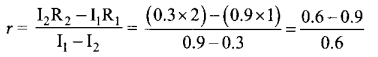|r| = |-0.5| Ω
Magnitude of internal resistance r = 0.5 Ω